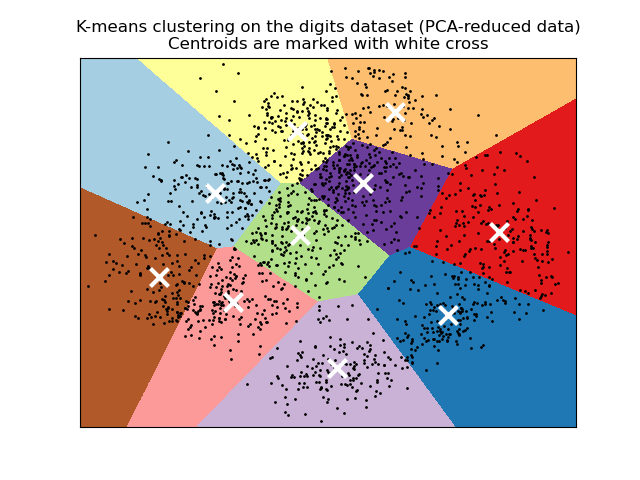# A demo of K-Means clustering on the handwritten digits data¶

In this example we compare the various initialization strategies for K-means in terms of runtime and quality of the results.

As the ground truth is known here, we also apply different cluster quality metrics to judge the goodness of fit of the cluster labels to the ground truth.

Cluster quality metrics evaluated (see Clustering performance evaluation for definitions and discussions of the metrics):

Shorthand

full name

homo

homogeneity score

compl

completeness score

v-meas

V measure

ARI

AMI

silhouette

silhouette coefficient

print(__doc__)


We will start by loading the digits dataset. This dataset contains handwritten digits from 0 to 9. In the context of clustering, one would like to group images such that the handwritten digits on the image are the same.

import numpy as np

(n_samples, n_features), n_digits = data.shape, np.unique(labels).size

print(
f"# digits: {n_digits}; # samples: {n_samples}; # features {n_features}"
)


Out:

# digits: 10; # samples: 1797; # features 64


## Define our evaluation benchmark¶

We will first our evaluation benchmark. During this benchmark, we intend to compare different initialization methods for KMeans. Our benchmark will:

• create a pipeline which will scale the data using a StandardScaler;

• train and time the pipeline fitting;

• measure the performance of the clustering obtained via different metrics.

from time import time
from sklearn import metrics
from sklearn.pipeline import make_pipeline
from sklearn.preprocessing import StandardScaler

def bench_k_means(kmeans, name, data, labels):
"""Benchmark to evaluate the KMeans initialization methods.

Parameters
----------
kmeans : KMeans instance
A :class:~sklearn.cluster.KMeans instance with the initialization
name : str
Name given to the strategy. It will be used to show the results in a
table.
data : ndarray of shape (n_samples, n_features)
The data to cluster.
labels : ndarray of shape (n_samples,)
The labels used to compute the clustering metrics which requires some
supervision.
"""
t0 = time()
estimator = make_pipeline(StandardScaler(), kmeans).fit(data)
fit_time = time() - t0
results = [name, fit_time, estimator[-1].inertia_]

# Define the metrics which require only the true labels and estimator
# labels
clustering_metrics = [
metrics.homogeneity_score,
metrics.completeness_score,
metrics.v_measure_score,
]
results += [m(labels, estimator[-1].labels_) for m in clustering_metrics]

# The silhouette score requires the full dataset
results += [
metrics.silhouette_score(data, estimator[-1].labels_,
metric="euclidean", sample_size=300,)
]

# Show the results
formatter_result = ("{:9s}\t{:.3f}s\t{:.0f}\t{:.3f}\t{:.3f}"
"\t{:.3f}\t{:.3f}\t{:.3f}\t{:.3f}")
print(formatter_result.format(*results))


## Run the benchmark¶

We will compare three approaches:

• an initialization using kmeans++. This method is stochastic and we will run the initialization 4 times;

• a random initialization. This method is stochastic as well and we will run the initialization 4 times;

• an initialization based on a PCA projection. Indeed, we will use the components of the PCA to initialize KMeans. This method is deterministic and a single initialization suffice.

from sklearn.cluster import KMeans
from sklearn.decomposition import PCA

print(82 * '_')
print('init\t\ttime\tinertia\thomo\tcompl\tv-meas\tARI\tAMI\tsilhouette')

kmeans = KMeans(init="k-means++", n_clusters=n_digits, n_init=4,
random_state=0)
bench_k_means(kmeans=kmeans, name="k-means++", data=data, labels=labels)

kmeans = KMeans(init="random", n_clusters=n_digits, n_init=4, random_state=0)
bench_k_means(kmeans=kmeans, name="random", data=data, labels=labels)

pca = PCA(n_components=n_digits).fit(data)
kmeans = KMeans(init=pca.components_, n_clusters=n_digits, n_init=1)
bench_k_means(kmeans=kmeans, name="PCA-based", data=data, labels=labels)

print(82 * '_')


Out:

__________________________________________________________________________________
init            time    inertia homo    compl   v-meas  ARI     AMI     silhouette
k-means++       0.086s  69662   0.680   0.719   0.699   0.570   0.695   0.173
random          0.072s  69707   0.675   0.716   0.694   0.560   0.691   0.188
PCA-based       0.023s  72686   0.636   0.658   0.647   0.521   0.643   0.144
__________________________________________________________________________________


## Visualize the results on PCA-reduced data¶

PCA allows to project the data from the original 64-dimensional space into a lower dimensional space. Subsequently, we can use PCA to project into a 2-dimensional space and plot the data and the clusters in this new space.

import matplotlib.pyplot as plt

reduced_data = PCA(n_components=2).fit_transform(data)
kmeans = KMeans(init="k-means++", n_clusters=n_digits, n_init=4)
kmeans.fit(reduced_data)

# Step size of the mesh. Decrease to increase the quality of the VQ.
h = .02     # point in the mesh [x_min, x_max]x[y_min, y_max].

# Plot the decision boundary. For that, we will assign a color to each
x_min, x_max = reduced_data[:, 0].min() - 1, reduced_data[:, 0].max() + 1
y_min, y_max = reduced_data[:, 1].min() - 1, reduced_data[:, 1].max() + 1
xx, yy = np.meshgrid(np.arange(x_min, x_max, h), np.arange(y_min, y_max, h))

# Obtain labels for each point in mesh. Use last trained model.
Z = kmeans.predict(np.c_[xx.ravel(), yy.ravel()])

# Put the result into a color plot
Z = Z.reshape(xx.shape)
plt.figure(1)
plt.clf()
plt.imshow(Z, interpolation="nearest",
extent=(xx.min(), xx.max(), yy.min(), yy.max()),
cmap=plt.cm.Paired, aspect="auto", origin="lower")

plt.plot(reduced_data[:, 0], reduced_data[:, 1], 'k.', markersize=2)
# Plot the centroids as a white X
centroids = kmeans.cluster_centers_
plt.scatter(centroids[:, 0], centroids[:, 1], marker="x", s=169, linewidths=3,
color="w", zorder=10)
plt.title("K-means clustering on the digits dataset (PCA-reduced data)\n"
"Centroids are marked with white cross")
plt.xlim(x_min, x_max)
plt.ylim(y_min, y_max)
plt.xticks(())
plt.yticks(())
plt.show()Total running time of the script: ( 0 minutes 2.641 seconds)

Gallery generated by Sphinx-Gallery